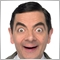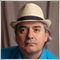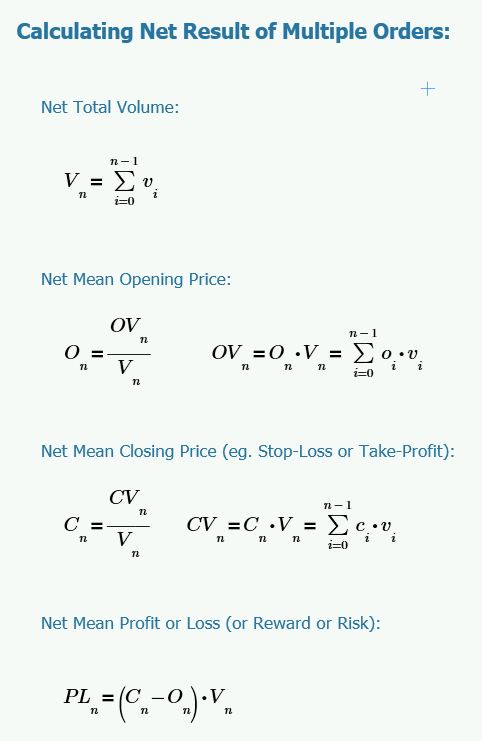# Average the entry price of the open trades86

hi guys its my first time coding mql4 and im still practicing. I have a problem on my project, I have 10 open trades on buy. i want to get the average of my entry price on buy, is that possible?Moderator
31778

mark692:
hi guys its my first time coding mql4 and im still practicing. I have a problem on my project, I have 10 open trades on buy. i want to get the average of my entry price on buy, is that possible?

Add all the entry prices together.

Divide by 10.86

Keith Watford:

Add all the entry prices together.

Divide by 10.

Thank you for responding sir Keith is there a function that will add all my entry price?Moderator
31778

```   double total=0;
for(int x=OrdersTotal()-1; x>=0; x--)
{
if(OrderSelect(x,SELECT_BY_POS))
total+=OrderOpenPrice();
}```86

Keith Watford:
Thank you so much i will try this :)Moderator
31778

mark692:
Thank you so much i will try this :)

You're welcome.7121

mark692: hi guys its my first time coding mql4 and im still practicing. I have a problem on my project, I have 10 open trades on buy. i want to get the average of my entry price on buy, is that possible?

Please note that if your orders have different volumes (lots) and you are trying to calculate the net open price (that will be equivalent to a single order for the entire batch), then you have to apply the following calculations:

Any great idea about HEDGING positions welcome here

Fernando Carreiro, 2018.09.17 21:17

For those of you that want to learn to see the "light" and not fall into the trap of "hedging" and Grid strategies, here is the basic math::

Learn to do your research properly! Do not be blinded by false promises. Do the math!86

Fernando Carreiro:

Please note that if your orders have different volumes (lots) and you are trying to calculate the net open price (that will be equivalent to a single order for the entire batch), then you have to apply the following calculations:

Hello sir Fernando thank you for your response, but your formula that you give is a bit confusing :(7121

mark692: Hello sir Fernando thank you for your response, but your formula that you give is a bit confusing :(

Well, it does require that you have some understanding of mathematics and its notation, in order to understand it. In essence:

1. You first sum the volume of all open positions (That is the first calculation for "Net Total Volume").
2. Then you sum the product of the open price and the volume of each position (that is to multiply the open price and volume for each position, and then sum it all up)
3. You then take the sum in step 2 and divide it by the sum of step one. That will give you the "Net Mean Opening Price".

You can apply the same logic in the calculation of the "Net Mean Closing Price" (or the S/L or the T/P).

For the "Net Mean Profit or Loss", subtract the "Net Mean Opening Price" (step 3) from the "Net Mean Closing Price", then multiple by the "Net Total Volume" (step 1).86

Fernando Carreiro:

Well, it does require that you have some understanding of mathematics and its notation, in order to understand it. In essence:

1. You first sum the volume of all open positions (That is the first calculation for "Net Total Volume").
2. Then you sum the product of the open price and the volume of each position (that is to multiply the open price and volume for each position, and then sum it all up)
3. You then take the sum in step 2 and divide it by the sum of step one. That will give you the "Net Mean Opening Price".

You can apply the same logic in the calculation of the "Net Mean Closing Price" (or the S/L or the T/P).

For the "Net Mean Profit or Loss", subtract the "Net Mean Opening Price" (step 3) from the "Net Mean Closing Price", then multiple by the "Net Total Volume" (step 1).

Sir Fernando is this what you mean volume? is that the lotsize?

im not good in english sorry7121

mark692: Sir Fernando is this what you mean volume? is that the lotsize? im not good in english sorry

Yes! Order "Volume" is measured it "Lots"!

The word "Mean" is equivalent to "Average"!